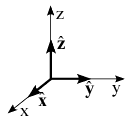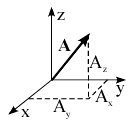$\def\xh{\hat{x}}$ $\def\yh{\hat{y}}$ $\def\zh{\hat{z}}$

When working with scalars, we have one definition of a product between scalars, one which gives another scalar. However, when working with vectors, we can envision several possibilities. For example, we can have a scalar product between two vectors which gives a scalar, or a vector product which gives another vector, or even a product which gives other mathematical entities (for the curious: called the outer product, and it gives a matrix, but we wont discuss that here!). In this section, we will be interested in the scaler product.

There are many possibilities which we can think of to define a scalar product between vectors. However, from all these possibilities, we wish to have a definition which is also useful! That is, we wish to have a definition which will satisfy several basic constrains we know are satisfied by the scalar product between scalars (i.e., the normal multiplication rules we know from grade school).

First and foremost, we wish the product $\star$ to be distributive, that is, that ${\bf A} \star ({\bf B}+{\bf C}) = {\bf A}\star {\bf B}+ {\bf A}\star {\bf C}$. An example for a definition which does not satisfy this constraint (and is therefore not very useful...) is:

$${\bf A} \star {\bf B} = |{\bf A}| |{\bf B}|.$$

To see that ${\bf A} \star ({\bf B}+{\bf C}) \neq {\bf A}\star {\bf B}+ {\bf A}\star {\bf C}$, we can look at the case ${\bf B} = - {\bf C}$. In such a case:

$${\bf A} \star ({\bf B} + {\bf C}) = 0$$

$${\bf A}\star {\bf B}+ {\bf A}\star {\bf C} = 2 |{\bf A}| |{\bf B}|$$

Thus, the above definition is rather useless. There is definition, however, which is very useful. It is the following.

The definition of the dot product (aka scalar product):

The dot product, also known as the scalar product $\cdot$" between two vectors ${\bf A}$ and ${\bf B}$ is defined as the size of ${\bf A}$ multiplied by the size of ${\bf B}$ and the cosine of the angle $\theta$ between them:

$${\bf A}\cdot {\bf B} \equiv |{\bf A}| |{\bf B}| \cos (\theta).$$

As we shall see below, this definition satisfies several useful constraints, which we will mention after we briefly dwell on the meaning of this product.

The meaning of the scalar product:

The figures below show us that the dot product between two vectors can be written as the following:

$${\bf A}\cdot {\bf B} \equiv |{\bf A}| |{\bf B}| \cos (\theta) = |{\bf A}| (|{\bf B}| \cos (\theta)) = |{\bf B}| (|{\bf A}| \cos (\theta))$$Namely, the dot product is the size of ${\bf A}$ times the size of the projection of ${\bf B}$ onto ${\bf A}$, or vice-versa, it is the size of ${\bf B}$ times the projection of the size of ${\bf A}$ onto ${\bf B}$.

Important characteristics of the dot product:

- The first point to note about the definition is that the coordinate system does not enter the definition.

- The second point to note is that because $\cos (\theta) = \cos (-\theta)$, the order is not important, that is, the scalar product is commutative:

$${\bf A}\cdot {\bf B} ={\bf B}\cdot {\bf A}.$$

- The scalar product is also distributive. To see this, we can look at the following diagram, which demonstrates that the projection of ${\bf A}+ {\bf B}$ onto ${\bf {\hat C}}$ is equal to the sum of the projection of ${\bf A}$ onto ${\bf {\hat C}}$ and the projection of ${\bf B}$ onto ${\bf \hat{C}}$. Thus, $({\bf A}+ {\bf B})\cdot {\bf {\hat C}} =({\bf A} \cdot {\bf {\hat C}})+({\bf B} \cdot {\bf {\hat C}})$. If we multiply each of the terms by $|{\bf C}|$, we find that $({\bf A}+ {\bf B})\cdot {\bf { C}} =({\bf A} \cdot {\bf { C}})+­ ({\bf B} \cdot {\bf {C}})$.Dot product of a vector with itself:

Since the angle between a vector and itself is zero, we have

$${\bf A}\cdot{\bf A} = |{\bf A}| |{\bf A}| \cos (0) = A^2$$

Namely, the dot product of a vector with itself gives its magnitude squared.

The opposite operation to the dot product:

With the scalar product between scalars we know that the opposite operation is the division. That is, if $a \times b = c$, we have that $a = c/b$. However, unlike the scalar equivalent, there is no meaning to the division in a vector. Alternatively, if we know ${\bf B}$ and we know ${\bf A}\cdot {\bf B}$, it is not possible to know ${\bf A}$ because there are many (infinite to be exact) possibilities for ${\bf A}$ – there is an infinite set of vectors which have the same projection.

Components of a vector in the cartesian coordinate system and the direction cosines:

The cartesian coordinate system is the simplest coordinate system possible. It is defined through three fixed and mutually orthogonal (i.e., perpendicular) directions. Often, they are denotes as the x, y and z directions.

Given the directions, we can define unit vectors in those directions: ${\bf \hat x}$, ${\bf \hat y}$ and ${\bf \hat z}$. Note that often an alternative notation is that of ${\bf \hat i}$, ${\bf \hat j}$ and ${\bf \hat k}$ instead. Both notations are equivalent.Since the aforementioned vectors are unit vectors, their length is 1:

$${\bf \hat x}\cdot {\bf \hat x }={\bf \hat y}\cdot {\bf \hat y }={\bf \hat z}\cdot {\bf \hat z }=1.$$

Since the vectors are perpendicular to each other, we have:

$${\bf \hat x}\cdot {\bf \hat y }={\bf \hat y}\cdot {\bf \hat z }={\bf \hat z}\cdot {\bf \hat x }=0.$$

Moreover, we can write each vector as:

$${\bf A} = A_x \xh + A_y \yh + A_z \zh$$.

What is the meaning of each term? To see this, let us look at the following:

$$({\bf A}\cdot \xh) = A_x (\underbrace{\xh \cdot \xh}_1) + A_y (\underbrace{\yh \cdot \xh}_{0}) + A_x (\underbrace{\zh \cdot \xh}_0) = A_x.$$

Thus, $A_x$ is the projection of ${\bf A}$ on the x-axis (i.e., the $\xh$ direction) times the size of $\xh$, which is unity. $A_x$ is therefore called the x component of the vector ${\bf A}$.Using the vector components, the dot product becomes:

$${\bf A} \cdot {\bf B} = (A_x \xh + A_y \yh +A_z \zh) \cdot (B_x \xh + B_y \yh +B_z \zh)$$ $$= A_x B_x (\underbrace{\xh \cdot \xh}_1) + A_x B_y (\underbrace{\xh \cdot \yh}_0) + ... = A_x B_x + A_y B_y + A_z B_z$$

That is, the dot product becomes the sum of the product of the respective components.

We can also relate the magnitude of ${\bf A}$ to its components:

$$A = \sqrt{{\bf A}\cdot {\bf A}} = \sqrt{(A_x \xh + A_y \yh +A_z \zh) \cdot (A_x \xh + A_y \yh +A_z \zh)}= \sqrt{A_x^2+A_y^2+A_z^2}.$$

Perpendicular vectors:

If $|{\bf A}| \neq 0$ and $|{\bf B}| \neq 0$, then the condition ${\bf A}\cdot {\bf B}=0$ implies that $\cos(\theta_{AB})=0$, that is, the angle $\theta_{AB}$ between the two vectors must be $\pi/2$ or $3\pi/2$. Hence, the vectors are perpendicular to each other.

­

## Blogroll

Sensible Climate Physics and more Other